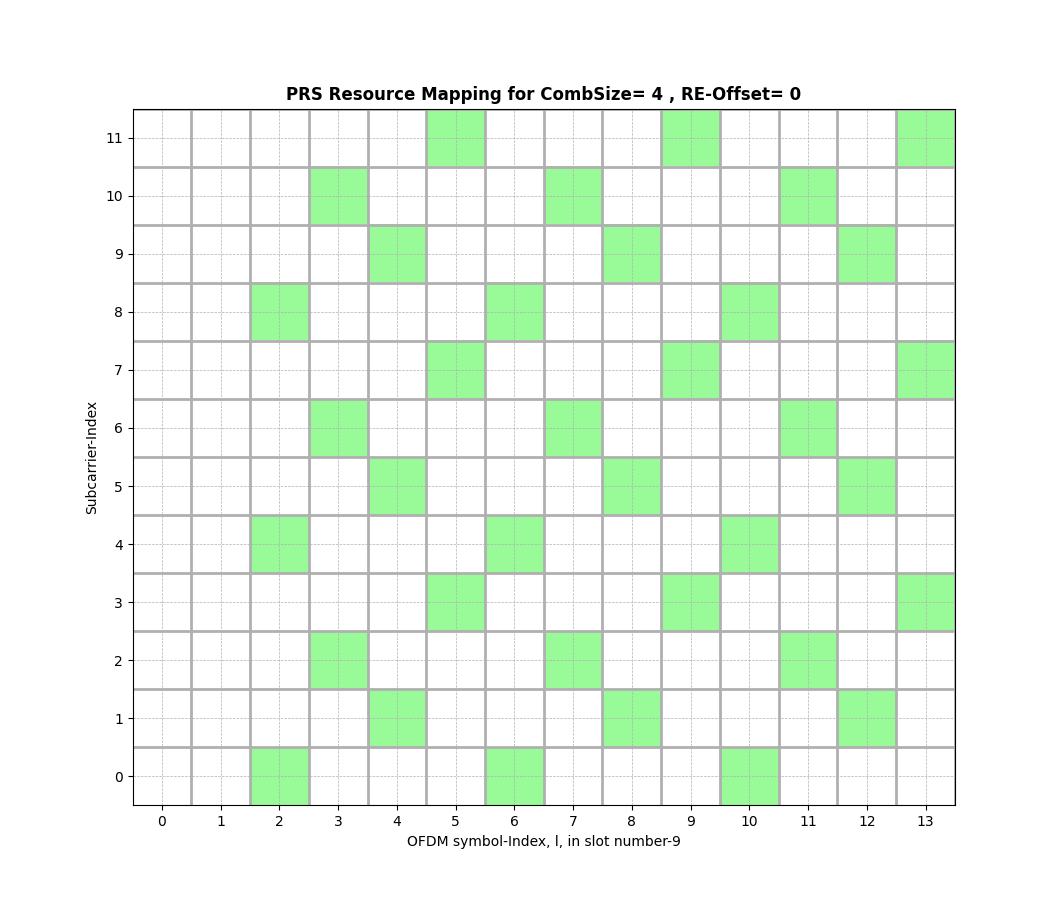# Positioning Reference Signal (PRS)

This module loads the positioning reference signal (PRS) in the resource grid based on the inputs configured. The details of the PRS resource mapping is provided in Section 7.4.1.7.3 of [3GPPTS38211_PRSRM].

Generating PRS Resource Grid:

subcarrierSpacing = 30000 # subcarrier spacing
numRBs            = 28    # number of Resource Grids

dl_PRS_NumSymbols           = 12   # Number of OFDM symbols occupied by PRS
dl_PRS_CombSizeN            = 4    # COMB size-N
dl_PRS_ResourceSymbolOffset = 2    # PRS symbol offset
dl_PRS_ReOffset             = 0    # RE offset in PRS
dl_PRS_SequenceID           = 1031 # PRS Sequence ID.

# Number of slots per frame
numSlotsPerFrame            = int(10*(subcarrierSpacing/15000))
slotNumber                  = 9    # Index of slot where the PRS is loaded
betaPRS                     = 1    # power scaling factor of PRS per ofdm symbol

# Object for generating the PRS Resource Grid
prsMapper = ResourceMapperPRS(betaPRS, dl_PRS_CombSizeN, dl_PRS_ReOffset,
dl_PRS_ResourceSymbolOffset, dl_PRS_NumSymbols, dl_PRS_SequenceID)

# Generate the PRS Resource Grid
prsGrid   = prsMapper(slotNumber, numRBs)


Display the PRS Resource Grid:

fig, ax = prsMapper.displayResourceGrid()class toolkit5G.ResourceMapping.ResourceMapperPRS(betaPRS, combSize, reOffset, startSymbol, numSymbols, sequenceID)[source]

Generates the resource grid for PRS as per Section 7.4.1.7 [3GPPTS38211_PRSRM].

Parameters:
• betaPRS (int) – Defines the PRS power scale factor. It should be a +ve value.

• combSize (int) – Defines the comb size $$\text{𝐾}_{\text{comb}} \in \{2, 4, 6,12\}$$ is given by the higher-layer parameter dl-PRS-CombSizeN-AndReOffset for a downlink PRS resource configured for RTT-based propagation delay compensation, otherwise by the higher-PRS layer parameter dl-PRS-CombSizeN.

• reOffset (int) – Defines the resource-element offset $$\text{k}_{\text{offset}} \in \{0,1,\dots,$$ $$\text{𝐾}_{\text{comb}}−1\}$$ is obtained from the higher-layer parameter dl-PRS- CombSizeN-AndReOffset;

• startSymbol (int) – Defines the $$\text{l}_{\text{start}}$$ is the first symbol of the downlink PRS within a slot and given by the higher-layer parameter dl-PRS-ResourceSymbolOffset.

• numSymbols (int) – Defines the size of the downlink PRS resource in the time domain $$\text{L}_{\text{PRS}} \in \{2,4,6,12\}$$ is given by the higher-layer parameter dl-PRS-NumSymbols;

• sequenceID (int) – Defines the dl-PRS-SequenceID $$\in \{0,1,\dots,4095\}$$.

Important

The numSymbols and combSize shall be selected from one of the combination $$\{2, 2\}$$, $$\{4, 2\}$$, $$\{6, 2\}$$, $$\{12,2\}$$, $$\{4, 4\}$$, $$\{12, 4\}$$, $$\{6, 6\}$$, $$\{12, 6\}$$ and $$\{12, 12\}$$.

Important

The numSymbols + startSymbol must be less than equal to 14.

Input:
• slotNumber (int) – Defines the slot number within a frame $$n_{s,f}^{\mu} \in \{0,1,2,$$ $$\dots,10*2^{\mu}-1\}$$ where $${\mu = \frac{\Delta f}{15 kHz}}$$ where PRS resource grid is loaded.

• numRBs (int) – Defines number of physical resource blocks (PRBs) occupied by PRS.

Warning

12*(startPRB + nrofPRBs) should be less than numSubcarrier.

Important

Size of frequencyDomainAllocation (N) can be 4 (for row 1 of Table 7.4.1.5.3-1), 12 (for row 2), 3 (for row 4), and 6 (for remaining rows).

Output:

[14, 12*numRBs], np.complex64 – Positioning Reference Signal (PRS) Grid.

Raises:
• ValueError – Invalid Length of frequencyDomainAllocation!

• ValueError – Invalid number of symbols configured for CSI-RS!

• ValueError – Error: Number of REs per symbols can’t be more than 2!

• ValueError – [Error in ResourceMapperCSIRS]: nrofPorts must be interger taking value from set {1,2,4,8,12,16,24,32}!

• ValueError – [Error in ResourceMapperCSIRS]: For nrofPorts = ” + str(self.__nrofPorts) + “, density must take value from set: ” + str(possibleDensity) + ” !”

• ValueError – [Error in ResourceMapperCSIRS]: For nrofPorts = ” + str(self.__nrofPorts) + ” and density = ” + str(self.__density) + “, cdmType must take value from set: ” + str(possibleCDMtype) + ” !”)

• ValueError – [Error in ResourceMapperCSIRS]: nrofPRBs must be a positive integer!”

• ValueError – [Error in ResourceMapperCSIRS]: startPRB must be a positive integer!”

• ValueError – [Error in ResourceMapperCSIRS]: numSubcarrier must be a positive integer!”

• ValueError – [Error in ResourceMapperCSIRS]: numSubcarrier is inconsistent with (startPRB, nrofPRBs)!”

• ValueError – [Error in ResourceMapperCSIRS]: rowIndex must be integer or integer array!”

• ValueError – [Error in ResourceMapperCSIRS]: rowIndex must take an integer from interval [1,18]!”

• ValueError – [Error in ResourceMapperCSIRS]: frequencyDomainAllocation must be integer or integer array!”

• ValueError – [Error in ResourceMapperCSIRS]: Elements of frequencyDomainAllocation must be 0 or 1!”

• ValueError – [Error in ResourceMapperCSIRS]: For rowIndex = ” + str(self.__rowIndex) + “, length of FrequencyDomainAllocation must be ” + str(numSCmap[self.__rowIndex]) + “!”)

• ValueError – [Error in ResourceMapperCSIRS]: firstOFDMSymbolInTimeDomain must be an integer from interval [0,13]!”

• ValueError – [Error in ResourceMapperCSIRS]: firstOFDMSymbolInTimeDomain2 must be passed for ‘Row’ = ” + str(self.__rowIndex) + “!”

• Warning – [Warning in ResourceMapperCSIRS]: firstOFDMSymbolInTimeDomain2 is redundant!”

• ValueError – [Error in ResourceMapperCSIRS]: firstOFDMSymbolInTimeDomain2 must be an integer from interval [2,12]!”

References:
[3GPPTS38211_PRSRM] (1,2)

(3GPP TS 38.211 version 17.1.0 Release 17) 5G;NR;Physical channels and modulation ‘5.2.2 Low-PAPR sequence generation type 1’